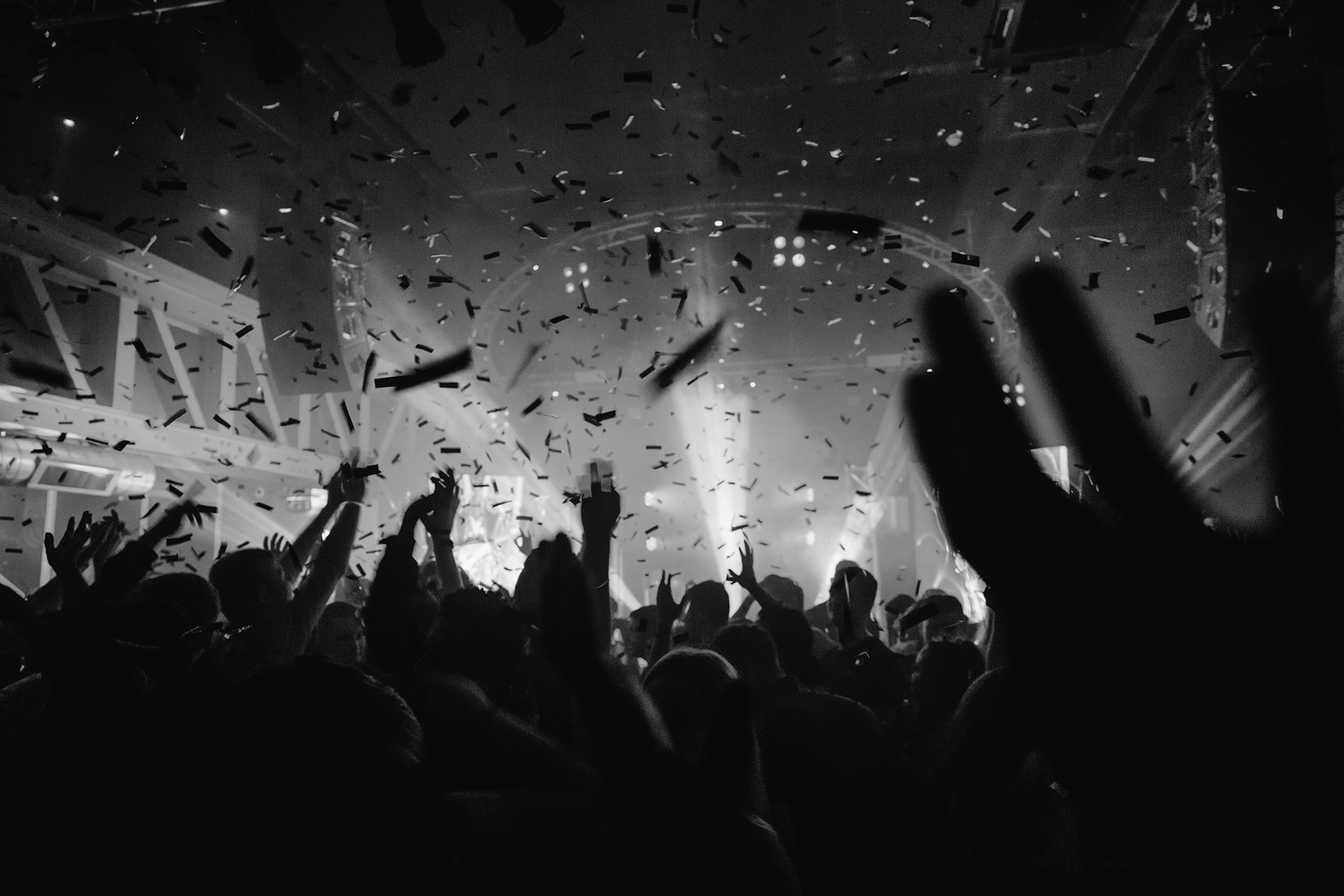B I G G E S T   |   B U S I E S T   |   B E S T

# T H E   G A R A G E

S I N C E   1 9 9 4

## E V E N T S

7  D A Y S   A   W E E K /  3 6 5   D A Y S   A   Y E A R

6  R O O M S   O V E R   4   F L O O R S

T H E   G A R A G E  |  G 2  |  T H E   J D   A T T I C   B A R

M A I N  B A R  |  D E S P E R A D O S  B A R C O C K T A I L  B A R